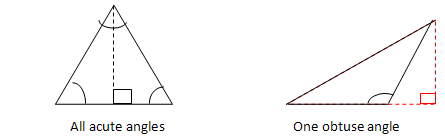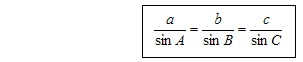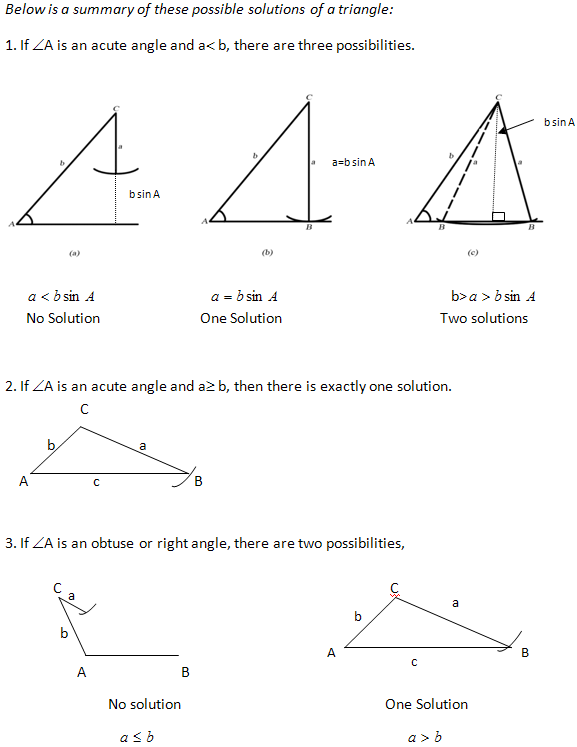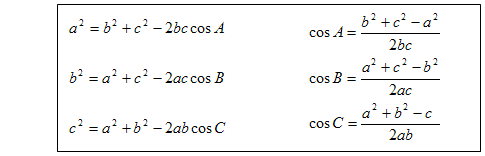# Trigonometry - Sine and Cosine Rule

## Introduction

The solution for an oblique triangle can be done with the application of the Law of Sine and Law of Cosine, simply called the Sine and Cosine Rules. An oblique triangle, as we all know, is a triangle with no right angle. It is a triangle whose angles are all acute or a triangle with one obtuse angle.

The two general forms of an oblique triangle are as shown:## Sine Rule (The Law of Sine)

The Sine Rule is used in the following cases:

CASE 1: Given two angles and one side (AAS or ASA)

CASE 2: Given two sides and a non-included angle (SSA)

The Sine Rule states that the sides of a triangle are proportional to the sines of the opposite angles. In symbols,## Case 2: SSA or The Ambiguous Case

In this case, there may be two triangles, one triangle, or no triangle with the given properties. For this reason, it is sometimes called the ambiguous case. Thus, we need to examine the possibility of no solution, one or two solutions.## Cosine Rule (The Law of Cosine)

The Cosine Rule is used in the following cases:

1. Given two sides and an included angle (SAS)

2. Given three sides (SSS)

The Cosine Rule states that the square of the length of any side of a triangle equals the sum of the squares of the length of the other sides minus twice their product multiplied by the cosine of their included angle. In symbols:Go to the next page to start practicing what you have learnt.